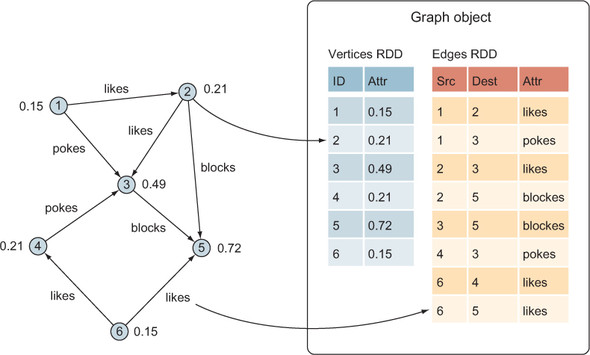# Chapter 4. GraphX Basics

### This chapter covers

• The basic GraphX classes
• The basic GraphX operations, based on Map/Reduce and Pregel
• Serialization to disk
• Stock graph generation

Now that we have covered the fundamentals of Spark and of graphs in general, we can put them together with GraphX. In this chapter you’ll use both the basic GraphX API and the alternative, and often better-performing, Pregel API. You’ll also read and write graphs, and for those times when you don’t have graph data handy, generate random graphs.

### 4.1. Vertex and edge classes

As discussed in chapter 3, Resilient Distributed Datasets (RDDs) are the fundamental building blocks of Spark programs, providing for both flexible, high--performance, data-parallel processing and fault-tolerance. The basic graph class in GraphX is called Graph, which contains two RDDs: one for edges and one for vertices (see figure 4.1).

##### Figure 4.1. A GraphX Graph object is composed of two RDDs: one for the vertices and one for the edges.One of the big advantages of GraphX over other graph processing systems and graph databases is its ability to treat the underlying data structures as both a graph, using graph concepts and processing primitives, and also as separate collections of edges and vertices that can be mapped, joined, and transformed using data-parallel processing primitives.